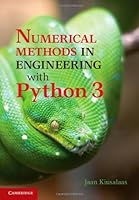# Numerical Methods in Engineering with Python 3, 3rd Edition## Book Description

This book is an introduction to numerical methods for students in engineering. It covers the usual topics found in an engineering course: solution of equations, interpolation and data fitting, solution of differential equations, eigenvalue problems, and optimization. The are implemented in 3, a high-level language that rivals ® in readability and ease of use. All methods include programs showing how the code is utilized in the solution of problems. The book is based on Numerical Methods in Engineering with Python, which used Python 2. This new text demonstrates the use of Python 3 and includes an introduction to the Python plotting package Matplotlib. This comprehensive book is enhanced by the addition of numerous examples and problems throughout.

Chapter 1 Introduction to Python
Chapter 2 Systems of Linear Algebraic Equations
Chapter 3 Interpolation and Curve Fitting
Chapter 4 Roots of Equations
Chapter 5 Numerical Differentiation
Chapter 6 Numerical Integration
Chapter 7 Initial Value Problems
Chapter 8 Two-Point Boundary Value Problems
Chapter 9 Symmetric Eigenvalue Problems
Chapter 10 Introduction to Optimization

## Book Details

• Title: Numerical Methods in Engineering with Python 3, 3rd Edition
• Author:
• Length: 432 pages
• Edition: 3
• Language: English
• Publisher:
• Publication Date: 2013-01-21
• ISBN-10: 1107033853
• ISBN-13: 9781107033856